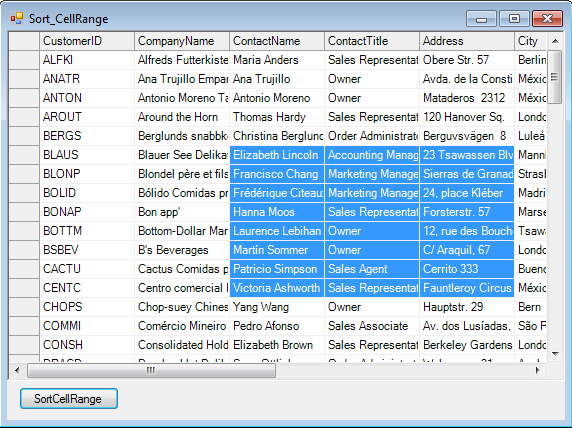There are occasions when users need to implement sort on a selected range of cells and not the entire C1Flexgrid. There's no direct method to implement this and here we will discuss implementation of the very same subject. Say there is a C1Flexgrid on the Form with a particular CellRange selected using Mouse or code and we need to sort that cell range. Lets drop a button on the Form besides the C1Flexgrid and start writing some code in the Click event of the button. And here's the logic: 1. Capture the CellRange object.

``````C1.Win.C1FlexGrid.CellRange cr = this.c1FlexGrid1.Selection;
``````

2. Create a temporary C1Flexgrid object.

``````var fg = new C1.Win.C1FlexGrid.C1FlexGrid();
``````
1. Add the same number of rows and columns to the temporary C1__flexgrid object as the number of rows and columns in CellRange.
``````fg.Cols.Count = cr.c2 - cr.c1 + 1;
fg.Cols.Fixed = 0;
fg.Rows.Count = cr.r2 - cr.r1 + 1;
fg.Rows.Fixed = 0;
``````
4. Populate this temporary ClFlexgrid with data from CellRange.
``````for (int i = cr.r1; i <= cr.r2; i++)
{
for (int j = cr.c1; j <= cr.c2; j++)
{
fg[\_row, \_col] = this.c1FlexGrid1[ i ,  j ];
_col += 1;
}
_row += 1;
_col = 0;
}
``````
5. Sort temporary C1Flexgrid.
``````fg.Sort(C1.Win.C1FlexGrid.SortFlags.Ascending, 0);
``````
6. Populate the sorted data from cells in temporary C1Flexgrid to the CellRange in original C1Flexgrid.
``````for (int i = cr.r1; i <= cr.r2; i++)
{
for (int j = cr.c1; j <= cr.c2; j++)
{
this.c1FlexGrid1[ i ,  j ] = fg[\_row, \_col];
_col += 1;
}
_row += 1;
_col = 0;
}
``````
Eventually the entire code in Button's Click event looks like: ~~~ //Get CellRange from selection done via Mouse
C1.Win.C1FlexGrid.CellRange cr = this.c1FlexGrid1.Selection;

//Create a temporary Flex object 'fg'.
var fg = new C1.Win.C1FlexGrid.C1FlexGrid();

//Set the same number of rows and columns in temp object as in the CellRange of the actual grid.
fg.Cols.Count = cr.c2 - cr.c1 + 1;
fg.Cols.Fixed = 0;
fg.Rows.Count = cr.r2 - cr.r1 + 1;
fg.Rows.Fixed = 0;

int _row = 0;
int _col = 0;

//Populate temp grid with data from selected range
for (int i = cr.r1; i <= cr.r2; i++)
{
for (int j = cr.c1; j <= cr.c2; j++)
{
fg[_row, _col] = this.c1FlexGrid1[ i , j ];
_col += 1;
}
_row += 1;
_col = 0;
}

//Set Sort Type and the column index you wish sort to be implemented on.
//In this case, leftmost column of 'fg', and thereby leftmost column
//of selected CellRange in actual grid gets sorted in Ascending order.
fg.Sort(C1.Win.C1FlexGrid.SortFlags.Ascending, 0);

_row = 0;
_col = 0;

//Populate the selected range in actual grid with data from temp grid.
for (int i = cr.r1; i <= cr.r2; i++)
{
for (int j = cr.c1; j <= cr.c2; j++)
{
this.c1FlexGrid1[i, j] = fg[_row, _col];
_col += 1;
}
_row += 1;
_col = 0;
}

//Dispose temporary Flexgrid Object
fg.Dispose(); ~~~ Here's a screenshot of a C1Flexgrid with a sorted CellRange.And we're done :) Download Sample